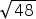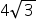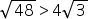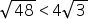Mathematics
Easy

Question

# Compareto## EquivalentNot equivalentHint:

## The correct answer is: Equivalent

### Here, we have to compare √48 to 4√3 .Now, √48 = √ ( 2 × 2 × 2 × 2 × 3 ) .= √ ( 24 × 3 ).= 22√3 i.e 4√3Clearly 4√3 is equal to 4√3 .Hence, the correct option is (a).

Two fractions are equal if they have the same value.

### Related Questions to study#### With Turito Foundation.#### Get an Expert Advice From Turito.